# Math in Focus Grade 3 Chapter 14 Practice 4 Answer Key Comparing Fractions

Practice the problems of Math in Focus Grade 3 Workbook Answer Key Chapter 14 Practice 4 Comparing Fractions to score better marks in the exam.

## Math in Focus Grade 3 Chapter 14 Practice 4 Answer Key Comparing Fractions

Compare the fractions.

Example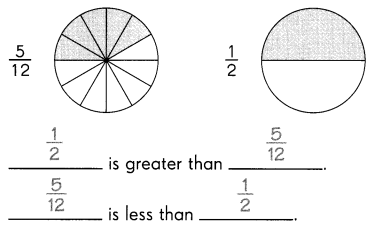Question 1.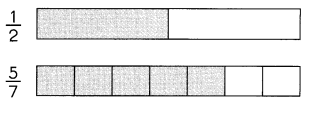___________ is greater than ___________.
5/7 is greater than 1/2.

Question 2.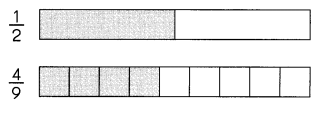___________ is less than ___________.
4/9 is less than 1/2.

Compare the fractions.

Question 3.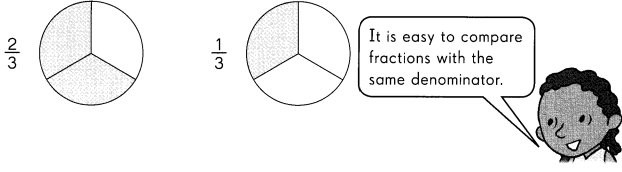___________ is greater than ___________.
2/3 is greater than 1/3.
Explanation:
In the above images we can observe two fractions 2/3 and 1/3. It is easy to compare fractions with the same denominator. The least fraction is the one with the lowest numerator. So, 2/3 is greater than 1/3.

Question 4.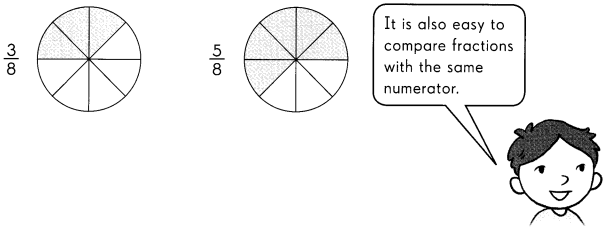___________ is less than ___________.
3/8 is less than 5/8.
Explanation:
In the above images we can observe two fractions 3/8 and 5/8. It is easy to compare fractions with the same denominator. The least fraction is the one with the lowest numerator. So, 3/8 is less than 5/8.

Question 5.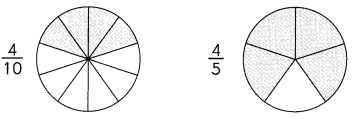___________ is greater than ___________.
4/5 is greater than 4/10.
Explanation:
In the above images we can observe two fractions 4/10 and 4/5. It is easy to compare fractions with the same numerator. The least fraction is the one with the greatest denominator. So, 4/5 is greater than 4/10.

Question 6.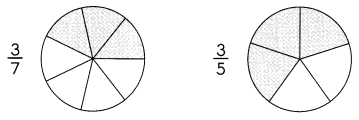___________ is less than ___________.
3/7 is less than 3/5.
Explanation:
In the above images we can observe two fractions 3/7 and 3/5. It is easy to compare fractions with the same numerator. The least fraction is the one with the greatest denominator. So, 3/7 is less than 3/5.

Compare the fractions.

Example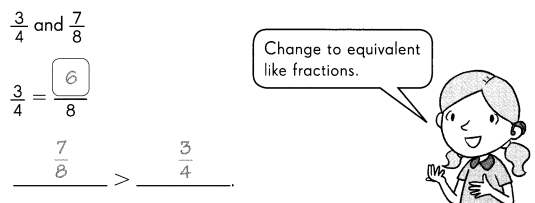Question 7.
$$\frac{7}{9}$$ and $$\frac{2}{3}$$
$$\frac{2}{3}$$ = __________
__________ < ___________
7/9 and 2/3
2/3 = 6/9
2/3 < 7/9
Explanation:
The equivalent fraction of 2/3 is 6/9. Compare 6/9 and 7/9. It is easy to compare fractions with the same denominator. The least fraction is the one with the lowest numerator. So, 2/3 is less than 7/9.

Question 8.
$$\frac{4}{5}$$ and $$\frac{1}{2}$$
$$\frac{4}{5}$$ = ____________
$$\frac{1}{2}$$ = ____________
__________ > __________
4/5 and 1/2
Below are the equivalent like fractions.
4/5 = 8/10
1/2 = 5/10
4/5 > 1/2
Explanation:
It is easy to compare fractions with the same denominator. The least fraction is the one with the lowest numerator. So, 4/5 is greater than 1/2.

Question 9.
$$\frac{5}{6}$$ and $$\frac{1}{4}$$
$$\frac{5}{6}$$ = ____________
$$\frac{1}{4}$$ = ____________
___________ < ___________
5/6 and 1/4
Below are the equivalent like fractions.
5/6 = 10/12
1/4 = 3/12
1/4 < 5/6
Explanation:
It is easy to compare fractions with the same denominator. The least fraction is the one with the lowest numerator. So, 1/4 is less than 5/6.

Use number lines to compare fractions.

Example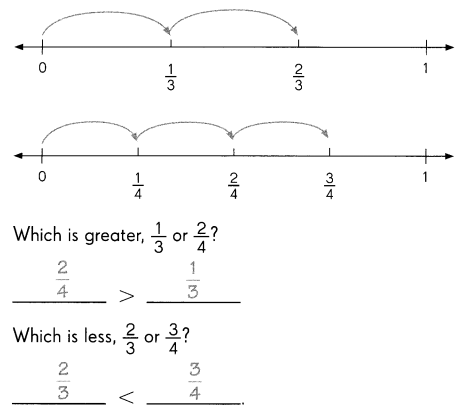Complete.

Question 10.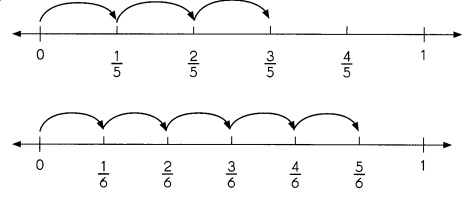Which is greater, $$\frac{3}{5}$$ or $$\frac{5}{6}$$?
___________ > __________
Which is less, $$\frac{2}{5}$$ or $$\frac{3}{6}$$?
___________ < __________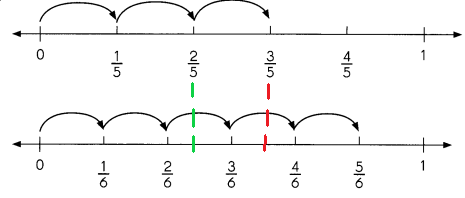5/6 > 3/5
2/5 < 3/6
Explanation:
In the above image we can observe two number lines.
Compare these two fractions 3/5 and 5/6. In first number line we can observe 3/5. In the second number line we can observe 5/6. The red line is drawn from first number line to second number line to know which fraction is greater. The fraction 5/6 is greater than 3/5.
Compare these two fractions 2/5 and 3/6. In first number line we can observe 2/5. In the second number line we can observe 3/6. The green line is drawn from first number line to second number line to know which fraction is less. The fraction 2/5 is less than 3/6.

Compare. Choose > or <. Use $$\frac{1}{2}$$ as a benchmark.

Question 11.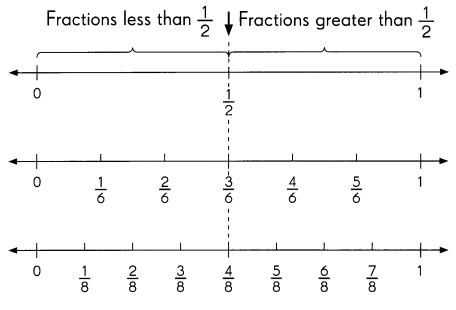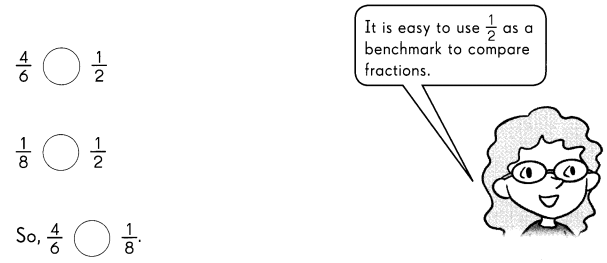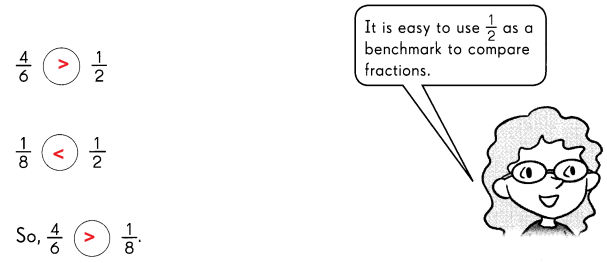Explanation:
In the above image we can observe three number lines. To compare the fractions we are using the first number line as 1/2 benchmark.
The fractions before 1/2 are the fractions less than 1/2.
The fractions after 1/2 are the fractions greater than 1/2.
The fraction 4/6 is greater than 1/2 as we can observe in the second number line.
The fraction 1/8 is less than 1/2 as we can observe in the third number line.
So, 4/6 is greater than 1/8.

Compare the fractions. Fill in the blanks.

Question 12.
$$\frac{4}{7}$$ and $$\frac{6}{7}$$
_____________ is greater.
4/7 and 6/7
6/7 is greater.
Explanation:
By comparing these two fractions 4/7 and 6/7. 6/7 is greater than 4/7.

Question 13.
$$\frac{2}{5}$$ and $$\frac{2}{10}$$
_____________ is greater.
2/5 and 2/10
2/5 is greater.
Explanation:
By comparing these two fractions 2/5 and 2/10. The simplified form of the fraction 2/10 is 1/5. So, 2/5 is greater than 2/10.

Question 14.
$$\frac{7}{12}$$ and $$\frac{1}{2}$$
_____________ is greater.
7/12 and 1/2
7/12 is greater.
Explanation:
By comparing these two fractions 7/12 and 1/2. The fraction 7/12 is greater than 1/2.

Question 15.
$$\frac{5}{11}$$ and $$\frac{1}{2}$$
_____________ is less.
5/11 and 1/2
5/11 is less.
Explanation:
By comparing these two fractions 5/11 and 1/2. The fraction 5/11 is less than 1/2.

Question 16.
$$\frac{7}{12}$$ and $$\frac{5}{11}$$
_____________ is less.
7/12 and 5/11
5/11 is less.
Explanation:
By comparing these two fractions 7/12 and 5/11. The fraction 5/11 is less than 7/12.

Order the fractions from greatest to least.

Example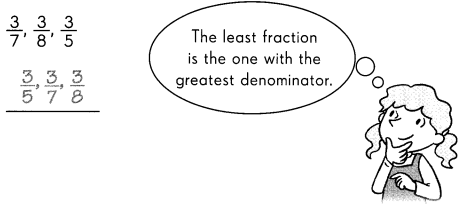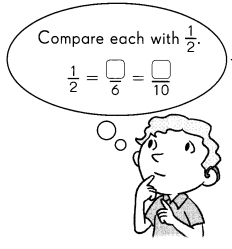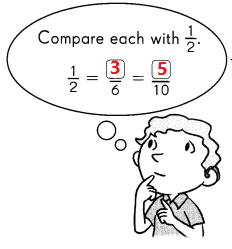Question 17.
$$\frac{7}{11}, \frac{5}{11}, \frac{11}{11}$$
11/11, 7/11, 5/11.
Explanation:
The least fraction is the one with the lowest numerator. The fractions from greatest to least are 11/11, 7/11, 5/11.

Question 18.
$$\frac{2}{3}, \frac{5}{6}, \frac{4}{10}$$
5/6, 2/3, 2/5
Explanation:
The fraction from greatest to least are 5/6, 2/3, 2/5.

Question 19.
$$\frac{1}{3}, \frac{1}{2}, \frac{3}{4}$$
$$\frac{1}{2}, \frac{2}{3}, \frac{3}{4}$$